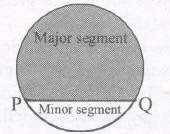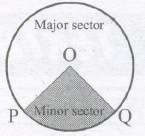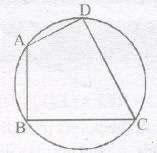# Circles Class 9 NCERT Notes For Class 9 Formulas Download PDF

Chapter 10 Circles circumference, Perimeter, Quality area of Circle formula

## Circles Notes For Class 9

### CIRCLES AND ITS RELATED TERMS

The collection of all the points in a plane, which are at a fixed distance from a fixed point in the plane, is called a circle.

A circle is a closed curve all of whose points lie in the same plane and are at the same distance from the centre.

The fixed point is called the centre of the circle and the fixed distance is called the radius of the circle.

Note : The line segment joining the centre and any point on the circle is also called a radius of the circle.

### INTERIOR AND EXTERIOR OF A CIRCLE

A circle divides the plane on which it lies into three parts. They are (i) inside the circle, which is also called the interior of the circle. (ii) the circle and (iii) outside the circle, which is also called the exterior of the circle

### CHORD

A chord of a circle is a line joining two points of the circumference. A chord passes through the centre is called diameter.

Note : Diameter is the longest chord and all diameters have the same length, which is equal to two times the radius.

A piece of a circle between two points is called an arc. In a circle equal chords have equal arcs.

When P and Q are ends of a diameter, then both arcs are equal and each is called a semicircle.

The length of the complete circle is called its circumference.

The region between a chord and either of its arcs is called segment of the circle.The region between an arc and the two radii, joining the centre to the end points of the arc is called a sector.

The minor arc corresponds to the minor sector and the major arc corresponds to the major sector.

When two arcs are equal, that is, each is a semicircle, then both segments and both sectors become the same and each is known as a semicircular region.Theorem 1: Equal chords of a circle subtend equal angles at the centre.

Theorem 2 : If the angles subtended by the chords of a circle at the centre are equal, then the chords are equal.

#### CHORD

Theorem 3 : The perpendicular from the centre of a circle to a chord bisects the chord.

Theorem 4 : The line drawn through the centre of a circle to bisect a chord is perpendicular to the chord.

Theorem 5 : There is one and only one circle passing through three given non-collinear points.

Remark : If ABC is a triangle, then by above given Theorem there is a unique circle passing through the three vertices A, B and C of the triangle. This circle is called the circumcircle of the AABC. Its centre and radius are called respectively the circumcentre and the circumradius of the triangle.

#### EQUAL CHORDS AND THEIR DISTANCES FROM THE CENTRE

Theorem 6 : Equal chords of a circle (or of congruent circles) are equidistant from the centre (or centres).

Theorem 7 : Chords equidistant from the centre of a circle are equal in length.

#### ANGLE SUBTENDED BY AN ARC OF A CIRCLE

Result : Congruent arcs (or equal arcs) of a circle subtend equal angles at the centre.

Theorem 8 :
The angle subtended by an arc at the centre is double the angle subtended by it at any point on the remaining part of the circle.

Note : Theorem gives the relationship between the angles subtended by an are at the centre and at a point on the circle.

#### ANGLE FORMED IN THE SEGMENT

Theorem 9:
Angles in the same segment of a circle are equal.

Note : Angle in a semicircle is a right angle.

Theorem 10 : If a line segment joining two points subtends equal angles at two other points lying on the same side of the line containing the line segment, the four points lie on a circle (i.e. they are concyclic).

A quadrilateral ABCD is called cyclic if all the four vertices of it lie on a circle.Theorem 11: The sum of either pair of opposite angles of a cyclic quadrilateral is 180°.

Theorem 12 : If the sum of a pair of opposite angles of a quadrilateral is 180°, the quadrilateral is cyclic.

### NCERT Books Free Pdf Download for Class 5, 6, 7, 8, 9, 10 , 11, 12 Hindi and English Medium

 Mathematics Biology Psychology Chemistry English Economics Sociology Hindi Business Studies Geography Science Political Science Statistics Physics Accountancy